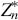## 2.2 ALGEBRA

### 2.2.1 Groups

Definition 2.10 A group (G, *, 1) consists of a set G with a binary operation * and an identity element 1 satisfying the following three axioms:

1.2.3. for each element x of G there exists an element x−1, called the inverse of x, such thatIf, furthermore,

4.(commutativity), the group is said to be commutative (or Abelian). Axioms 1 and 2 define a semigroup.

Examples 2.6

(Z, +, 0), (Zn, +, 0), (, ., 1)

The following definitions generalize Definitions 2.9.

Definitions 2.11

1. The order of an element x of a finite group G is the least positive integer t such that2. If the order of x is equal to the number n of elements in G, then x is said to be a generator of G.
3. If G has a generator, then G is said to be cyclic.

Property 2.6 The order of an element x of a finite group G divides the number of elements in G..

Proof First observe that if H is a subgroup of G, then an equivalence ...

Get Synthesis of Arithmetic Circuits: FPGA, ASIC and Embedded Systems now with the O’Reilly learning platform.

O’Reilly members experience books, live events, courses curated by job role, and more from O’Reilly and nearly 200 top publishers.